• 二阶椭圆偏微分方程实例求解(附matlab代码).docx 《微分方程数值解法》期中作业实验报告二阶椭圆偏微分方程第一边值问题姓名：学号：班级：2013...椭圆偏微分方程第一边值问题摘要对于解二阶椭圆偏微分方程第一边值问题...二阶椭圆偏微分方程实例求解(附matlab代码).docx 《微分方程数值解法》期中作业实验报告二阶椭圆偏微分方程第一边值问题姓名：学号：班级：2013年11月19日1二阶椭圆偏微分方程第一边值问题摘要对于解二阶椭圆偏微分方程第一边值问题，课本上已经给出了相应的差分方程。而留给我的难题就是把差分方程组表示成系数矩阵的形式，以及对系数进行赋值。解决完这个问题之后，我在利用matlab解线性方程组时，又出现“outofmemory”的问题。因为99*99阶的矩阵太大，超出了分配给matlab的使用内存。退而求其次，当n=10，h=1/10或n=70，h=1/70时，我都得出了很好的计算结果。然而在解线性方程组时，无论是LU分解法或高斯消去法，还是gauseidel迭代法，都能达到很高的精度。关键字：二阶椭圆偏微分方程差分方程outofmemoryLU分解高斯消去法gauseidel迭代法一、题目重述解微分方程：22(,)(,)()(,)(,(,)1yxxyyxyyeueuuuxye已知边界:(0,),(),(0),(1)xe==求数值解,把区域分成，n=100[]G´2/,/0h注：老师你给的题F好像写错了，应该把改成。xye2yxe二、问题分析与模型建立2.1微分方程上的符号说明𝐴(𝑥,𝑦)=𝑒𝑦𝐵(𝑥,𝑦)=𝑒𝑥𝐶(𝑥,𝑦)=𝑥+𝑦𝐷(𝑥,𝑦)=𝑥‒𝑦𝐸(𝑥,𝑦)=1𝐹(𝑥,𝑦)=221yxyxyee2.2课本上差分方程的缺陷课本上的差分方程为：𝑎𝑖𝑗𝑢𝑖𝑗‒(𝑎𝑖‒1,𝑗𝑢𝑖‒1,𝑗+𝑎𝑖,𝑗‒1𝑢𝑖,𝑗‒1+𝑎𝑖+1,𝑗𝑢𝑖+1,𝑗+𝑎𝑖,𝑗+1𝑢𝑖,𝑗+1)=𝐹𝑖𝑗2{𝑎𝑖‒1,𝑗=ℎ‒2(𝐴𝑖‒1/2,𝑗+ℎ𝐶𝑖𝑗2)𝑎𝑖,𝑗‒1=ℎ‒2(𝐵𝑖,𝑗‒1/2+ℎ𝐷𝑖𝑗2)𝑎𝑖+1,𝑗=ℎ‒2(𝐴𝑖+1/2,𝑗‒ℎ𝐶𝑖𝑗2)𝑎𝑖,𝑗+1=ℎ‒2(𝐵𝑖,𝑗+1/2‒ℎ𝐷𝑖𝑗2)𝑎𝑖𝑗=ℎ‒2(𝐴𝑖+1/2,𝑗+𝐴𝑖‒1/2,𝑗+𝐵𝑖,𝑗‒1/2+𝐵𝑖,𝑗+1/2)+𝐸𝑖𝑗 举一个例子：当i=2,j=3时，；当i=3,j=3时，。但𝑎𝑖𝑗=𝑎23𝑎𝑖‒1，𝑗=𝑎23是，显然这两个不是同一个数，其大小也不相等。𝑎232.3差分方程的重新定义因此，为了避免2.2中赋值重复而产生的错误，我在利用matlab编程时，对这些系数变量进行了重新定义：𝑏𝑖𝑗=𝑎𝑖𝑗，𝑐𝑖𝑗=𝑎𝑖,𝑗+1，𝑑𝑖𝑗=𝑎𝑖,𝑗‒1，𝑔𝑖𝑗=𝑎𝑖+1,𝑗，𝑘𝑖𝑗=𝑎𝑖‒1,𝑗.2.4模型建立这里的模型建立就是把差分方程组改写成系数矩阵的形式。经过研究，我觉得写成如下的系数矩阵不仅看起来简单明了，而且在matlab编程时比较方便。系数矩阵为：Pu=f其中P是阶方阵，具体如下：(𝑛‒1)2(𝑏11𝑐110𝑑12𝑏12⋱0⋱⋱00⋱𝑐1,𝑛‒2𝑑1,𝑛‒1𝑏1,𝑛‒1𝑔11𝑔12𝑔13⋱𝑔1,𝑛‒1𝑘21𝑘22𝑘2,3⋱𝑘2,𝑛‒1𝑏21𝑐210𝑑22𝑏22⋱0⋱⋱00⋱𝑐2,𝑛‒2𝑑1,𝑛‒1𝑏2,𝑛‒100⋱𝑔𝑛‒2,1𝑔𝑛‒2,2𝑔𝑛‒23⋱𝑔𝑛‒2,,𝑛‒1𝑘𝑛‒1,1𝑘𝑛‒1,2𝑘𝑛‒1,3⋱𝑘𝑛‒1,𝑛‒1𝑏𝑛‒1,1𝑐𝑛‒1,10𝑑𝑛‒1,2𝑏𝑛‒1,2⋱0⋱⋱00⋱𝑐𝑛‒1,𝑛‒2𝑑𝑛‒1,𝑛‒1𝑏𝑛‒1,𝑛‒1)3而u是维的列向量，具体如下：(𝑛‒1)2u=(𝑢11𝑢12⋮𝑢1,𝑛‒1𝑢21⋮⋮𝑢𝑛‒1,𝑛‒1)而f是维的列向量，具体如下：(𝑛‒1)2𝑓=(𝑓11𝑓12⋮𝑓1,𝑛‒1𝑓21⋮⋮𝑓𝑛‒1,𝑛‒1)三、求解过程3.1对系数矩阵的分析对上述模型的求解就是对线性方程组的求解。通过观察，我发现P是一个对角占优的矩阵，这不仅确定了解的唯一性，还保证了迭代法的收敛性。此外，还可以确定进行LU分解，若使用高斯消去法还可以省去选主元的工作。3.2matlab编程因为矩阵阶数过大，所以此题的编程难点为构造系数矩阵，即对线性方程组的赋值。我采用的方法是分块赋值。对于P的赋值，过程如下：第一步：𝑏𝑐𝑑𝑖=(𝑏𝑖1‒𝑐𝑖10‒𝑑𝑖2𝑏𝑖2⋱0⋱⋱00⋱‒𝑐𝑖,𝑛‒2‒𝑑𝑖,𝑛‒1𝑏𝑖,𝑛‒1),𝑔𝑖=[𝑔𝑖1𝑔𝑖2⋮𝑔𝑖,𝑛‒1],𝑘𝑖=[𝑘𝑖1𝑘𝑖2⋮𝑘𝑖,𝑛‒1]4第二步：𝐵𝐶𝐷=(𝑏𝑐𝑑1𝑏𝑐𝑑200⋱𝑏𝑐𝑑𝑖),𝐺=[𝑔1𝑔2⋮𝑔𝑛‒2],𝐾=[𝑘2𝑘3⋮𝑘𝑛‒1]第三步：P=BCD-diag(G,99)-diag(K,99).P和f的具体构造见附录6.1主代码3.3编程求解过程中的问题3.3.1问题产生当按照老师要求，n=100，h=1/100时，运行编好的matlab程序时，会出现如图3.1的错误提示。图3.13.3.2问题分析在matlab的命令窗口输入“memory”，出现如图3.2的内存使用情况，可以得出：MemoryusedbyMATLAB:454MB(4.760e+008bytes)。，若不用稀疏矩阵定义P，经过粗略计算，我发现矩阵P就要占800MB左右的内存，加上其他数据，内存消耗至少在1G以上。但是我电脑上分配给matlab的内存只有：454MB，即使在关闭杀毒软件等大部分应用程序后，分配给matlab的内存也刚够1G。图3.23.3.3问题解决经过上网查找资料后，我找到了如下几个解决方法。1)尽量早的分配大的矩阵变量2)尽量避免产生大的瞬时变量，当它们不用的时候应该及时clear。3)尽量的重复使用变量(跟不用的clear掉一个意思)4)将矩阵转化成稀疏形式5)使用pack命令56)如果可行的话，将一个大的矩阵划分为几个小的矩阵，这样每一次使用的内存减少。7)增大内存针对本题，我觉得比较理想的解决方法是采用稀疏矩阵的方式定义P。这样可以有效的减小P的内存消耗。但是考虑到老师的这次期中作业主要是考察我们对二阶椭圆偏微分方程的理解与实例操作，而不是旨在考察我们的matlab编程能力。因此我在此，略作偷懒，把n改成10或70(75以上内存就不够用了)，适当的降低精度来得到结果。四、计算结果4.1当n=10,h=1/10时的结果取n=10,h=1/10时，matlab运行的部分结果如图4.1。表4.2为LU分解法和高斯消去法的部分结果(这两个直接法结果完全一样)，表4.3为迭代法的部分结果。图4.1i,j数值解真实值误差1，11.0100501453351.0100501670840.0000000217491，21.0202012644381.0202013400270.0000000
展开全文• 最近在做毕业设计，自己的控制对象描述形式是传递函数，而书里看到的大部份被控对象都是差分方程形式，就想有没有这种转换的方法，网上搜索了一圈，总结如下。 步骤一，先建立传递函数方程 首先给定一个连续的传递...
最近在做毕业设计，自己的控制对象描述形式是传递函数，而书里看到的大部份被控对象都是差分方程的形式，就想有没有这种转换的方法，网上搜索了一圈，总结如下。
步骤一，先建立传递函数方程
首先给定一个连续的传递函数，例如一个二阶传递函数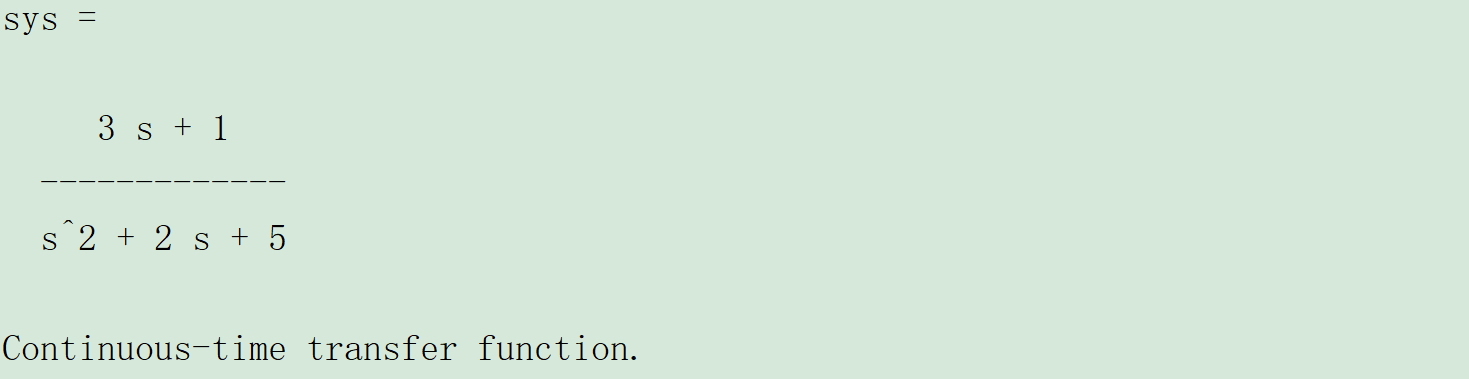在MATLAB里面的描述如下：
sys=tf([3,1],[1,2,5]);       % 建立被控对象传递函数

步骤二：将传递函数离散化
将传递函数离散成如下z函数的形式：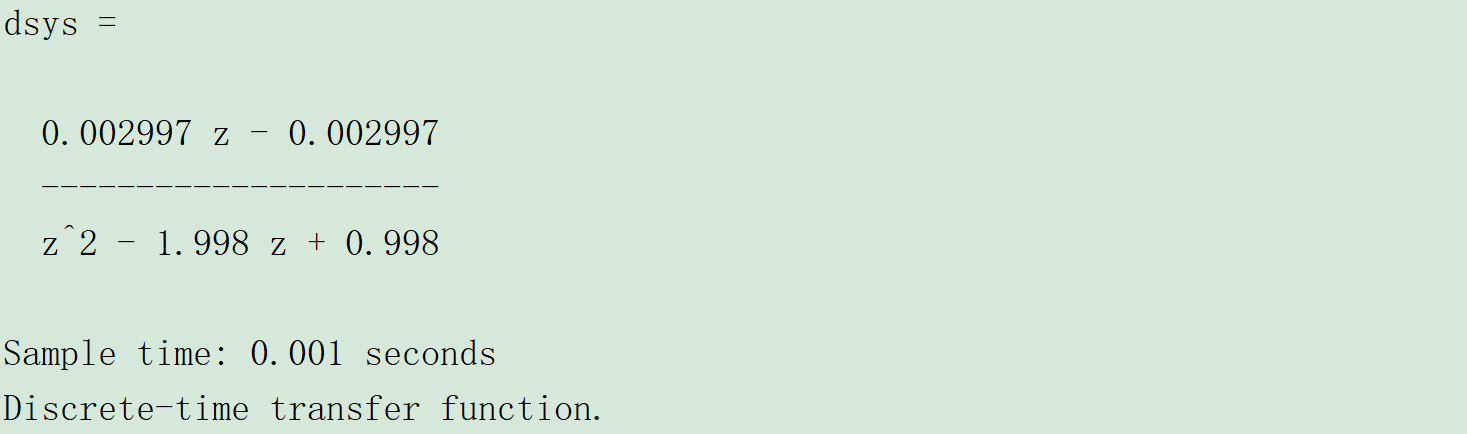这时就得到了一个离散模型，在MATLAB中描述如下：
ts=0.001;                      % 设定采样时间=0.001s
dsys=c2d(sys,ts,'z');          % 根据采样时间，把传递函数离散化

步骤三：把离散方程改成差分方程

模型改写成差分方程 首先把dsys改成y(z)和u(z)的比值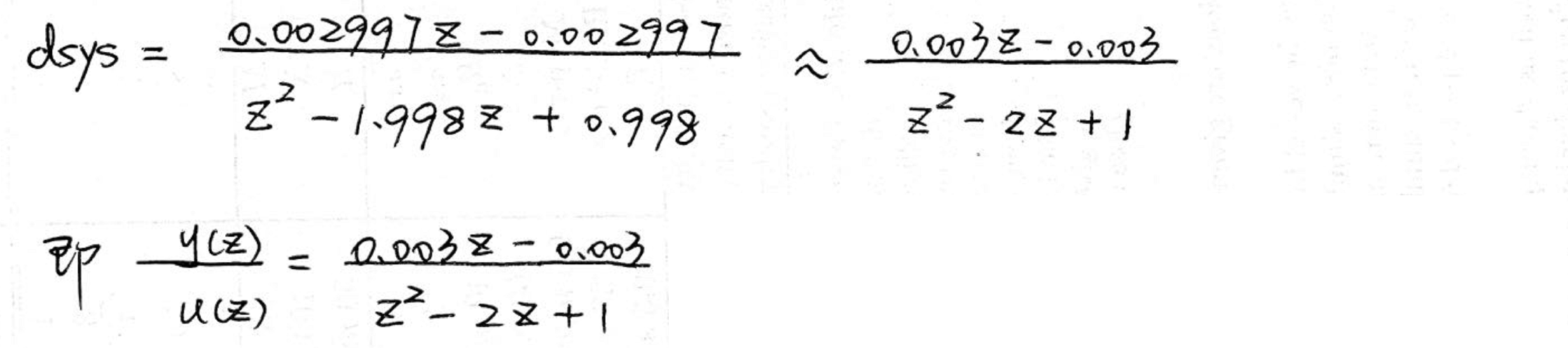然后上下同除以z的平方，使z的次数为负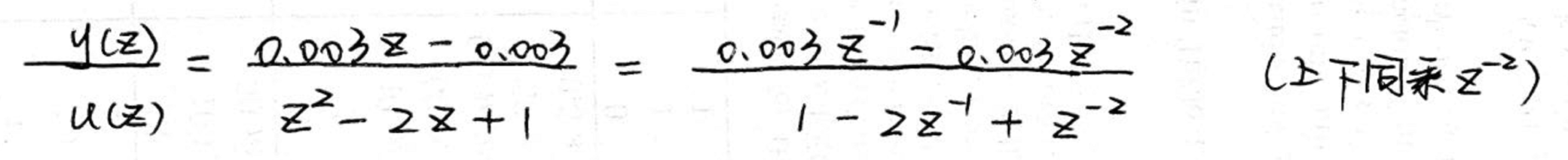接着十字相乘，展开成等式，并将z的幂次形式改写成差分形式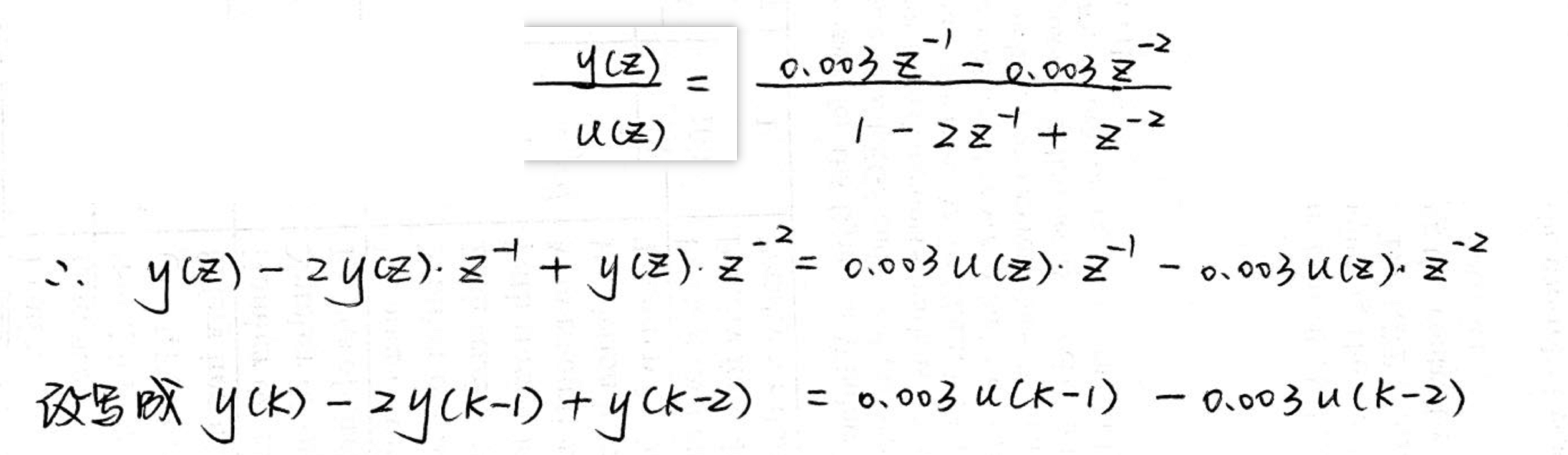最后把y(k)提到等式的左边，即得到差分方程的形式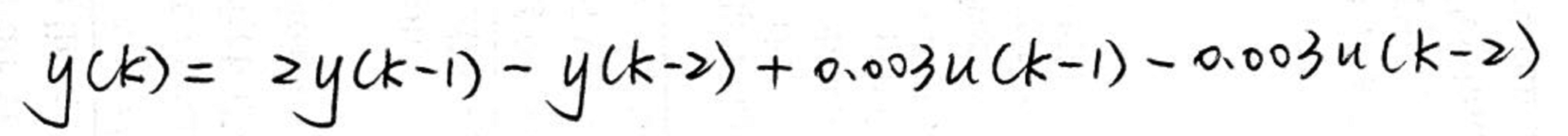展开全文MATLAB 传递函数
• 有限差分法以Taylor级数展开等方法，把控制方程中的导数用网格节点上的函数值的差商代替进行离散，从而建立以网格节点上的值为未知数的代数方程组。该方法是一种直接将微分问题变为代数问题的近似数值解法，数学概念...
有限差分法
有限差分方法(FDM)是计算机数值模拟最早采用的方法，至今仍被广泛运用。
该方法将求解域划分为差分网格，用有限个网格节点代替连续的求解域。有限差分法以Taylor级数展开等方法，把控制方程中的导数用网格节点上的函数值的差商代替进行离散，从而建立以网格节点上的值为未知数的代数方程组。该方法是一种直接将微分问题变为代数问题的近似数值解法，数学概念直观，表达简单，是发展较早且比较成熟的数值方法。
分类
对于有限差分格式，从格式的精度来划分，有一阶格式、二阶格式和高阶格式。从差分的空间形式来考虑，可分为中心格式和逆风格式。考虑时间因子的影响，差分格式还可以分为显格式、隐格式、显隐交替格式等。目前常见的差分格式，主要是上述几种形式的组合，不同的组合构成不同的差分格式。差分方法主要适用于有结构网格，网格的步长一般根据实际情况和条件来决定。
构造差分的方法
构造差分的方法有多种形式，目前主要采用的是泰勒级数展开方法。其基本的差分表达式主要有三种形式：一阶向前差分、一阶向后差分、一阶中心差分和二阶中心差分等，其中前两种格式为一阶计算精度，后两种格式为二阶计算精度。通过对时间和空间这几种不同差分格式的组合，可以组合成不同的差分计算格式。
泰勒级数：Python中调用sympy模块求解差分
from sympy import diff
from sympy import symbols
import sympy
def func(t):
return 2000 * sympy.log(14*10000/(14*10000-2100*t))-9.8*t  #函数
t = symbols("t")
print(func(16))
print(diff(func(t),t))  #对函数进行求导
print(diff(func(t),t).subs(t,16))

运行上述程序结果：
392.073691403521
588000000000*(1 - 3*t/200)/(140000 - 2100*t)**2 - 9.8
29.6736842105263

【注意】：
函数的写法不能调用sympy以外的模块的函数
比如这样写就不对：
from sympy import diff
from sympy import symbols
import math
def func(t):
return 2000 * math.log(14*10000/(14*10000-2100*t))-9.8*t
t = symbols("t")
print(func(16))
print(diff(func(t),t))
print(diff(func(t),t).subs(t,16))

结果：
392.07369140352074
print(diff(func(t),t))
return 2000 * math.log(14*10000/(14*10000-2100*t))-9.8*t
raise TypeError("can't convert expression to float")
TypeError: can't convert expression to float

错误点在于：sympy的模块的函数不能调用math模块的函数
一阶向前差分Python代码：
import sympy
from sympy import diff
from sympy import symbols

#差分的对象
x = 16
k = 2  #步长
x1 = x + k  #向前
x2 = x - k  #向后

#方程式
def func(t):
return 2000 * sympy.log(14*10000/(14*10000-2100*t))-9.8*t

#一阶向前差分
def for_difference():
a_for_diff = (func(x1) - func(x))/k
for_error = abs(a_for_diff - a_true)/a_true
print(f'{x}的一阶向前差分值：{a_for_diff}')
print(f'{x}的一阶向前差分的误差：{for_error*100}%')

if __name__ == '__main__':
t = symbols("t")
a_true = diff(func(t), t).subs(t, x)  # 真值
for_difference()

结果：
16的一阶向前差分值：30.4738991379398
16的一阶向前差分的误差：2.69671578943888%

一阶向后差分Python代码：
import sympy
from sympy import diff
from sympy import symbols

#差分的对象
x = 16
k = 2  #步长
x1 = x + k  #向前
x2 = x - k  #向后

#方程式
def func(t):
return 2000 * sympy.log(14*10000/(14*10000-2100*t))-9.8*t

#一阶向后差分
def beh_difference():
a_beh_diff = (func(x) - func(x2))/k
beh_error = abs(a_beh_diff - a_true)/a_true
print(f'{x}的一阶向后差分值：{a_beh_diff}')
print(f'{x}的一阶向后差分的误差：{beh_error*100}%')

if __name__ == '__main__':
t = symbols("t")
a_true = diff(func(t), t).subs(t, x)  # 真值
beh_difference()

运行结果：
16的一阶向后差分值：28.9145121806904
16的一阶向后差分的误差：2.55840166138381%

一阶中心差分Python代码：
import sympy
from sympy import diff
from sympy import symbols

#差分的对象
x = 16
k = 2  #步长
x1 = x + k  #向前
x2 = x - k  #向后

#方程式
def func(t):
return 2000 * sympy.log(14*10000/(14*10000-2100*t))-9.8*t

#一阶中心差分
def cen_difference():
a_cen_diff = (func(x1)-func(x2))/(k*2)
cen_error = abs(a_cen_diff - a_true)/a_true
print(f'{x}的二阶中心差分值：{a_cen_diff}')
print(f'{x}的二阶中心差分的误差：{cen_error*100}%')

if __name__ == '__main__':
t = symbols("t")
a_true = diff(func(t), t).subs(t, x)  # 真值
cen_difference()

代码运行结果：
16的二阶中心差分值：29.6942056593151
16的二阶中心差分的误差：0.0691570640275347%

二阶中心差分Python代码：
import sympy
from sympy import diff
from sympy import symbols

#差分的对象
x = 16
k = 2  #步长
x1 = x + k  #向前
x2 = x - k  #向后

#方程式
def func(t):
return 2000 * sympy.log(14*10000/(14*10000-2100*t))-9.8*t

#二阶中心差分
def two_cen_difference():
a_cen_diff = (func(x1)+func(x2)-2*func(x))/(k**2)
cen_error = abs(a_cen_diff - a_true)/a_true
print(f'{x}的二阶中心差分值：{a_cen_diff}')
print(f'{x}的二阶中心差分的误差：{cen_error*100}%')

if __name__ == '__main__':
t = symbols("t")
a_true = diff(func(t), t , 2).subs(t, x)  # 求2阶导真值
two_cen_difference()

程序运行结果：
16的二阶中心差分值：0.779693478624694
16的二阶中心差分的误差：0.0779896119162236%

迭代直到精确要求——以一阶向前差分为例
要求初始步长为2，经过多次迭代，一阶向前差分的误差能小于1%
Python代码如下：
import sympy
from sympy import diff
from sympy import symbols

#方程式
def func(t):
return 2000 * sympy.log(14*10000/(14*10000-2100*t))-9.8*t

#一阶向前差分
def for_difference(x1,x,a_true,k):
a_for_diff = (func(x1) - func(x))/k
for_error = abs(a_for_diff - a_true)/a_true
print(f'{x}的一阶向前差分值：{a_for_diff}')
print(f'{x}的一阶向前差分的误差：{for_error*100}%')
return for_error

def Judge_precision(x):
D = True
k = 2  # 初始步长
n = 0
while D:
n += 1
x1 = x + k  # 向前
t = symbols("t")
a_true = diff(func(t), t).subs(t, x)
error = for_difference(x1,x,a_true,k)
if error <= 0.01:
D = False
print(f'迭代第{n}次后，{x}的一阶向前差分的误差为{error}')
else:
k = k/2

if __name__ == '__main__':
x = 16  #差分对象
Judge_precision(x)

运行结果：
16的一阶向前差分值：30.4738991379398
16的一阶向前差分的误差：2.69671578943888%
16的一阶向前差分值：30.0684298016344
16的一阶向前差分的误差：1.33028844112341%
16的一阶向前差分值：29.8697466293837
16的一阶向前差分的误差：0.660728265039121%
迭代第3次后，16的一阶向前差分的误差为0.00660728265039121



展开全文python 算法
• 之前我们讲过一阶滤波器，思路就是把一个连续的滤波器形式，通过离散化的方式，转换成差分方程。 同事拿着我的文章，对照着代码里的二阶滤波，表示完全看不懂，我说不可能，二阶不过是一阶的升级版，思路应是一样的...
之前我们讲过一阶滤波器，思路就是把一个连续的滤波器形式，通过离散化的方式，转换成差分方程。
同事拿着我的文章，对照着代码里的二阶滤波，表示完全看不懂，我说不可能，二阶不过是一阶的升级版，思路应是一样的，他说不信你看。
我一看，WTF，这系数怎么来的？经验公式？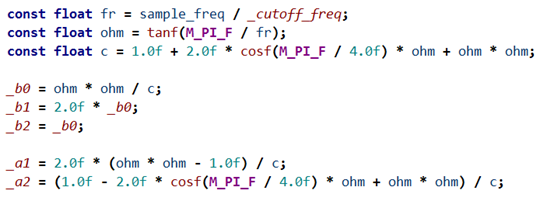这迭代怎么这种形式？没见过呀！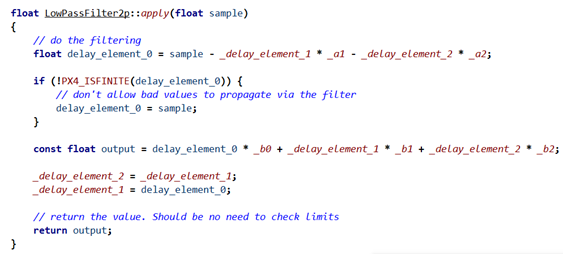行吧，说明之前咱理解的不到位，那就从头开始讲起吧。
从模拟滤波器开始
我们从书上，百度，查到的滤波器公式，通常是用传递函数表达的，这是s域下的表达形式，是连续的，这种我们称之为模拟滤波器。
以巴特沃斯低通滤波器为例：
$H_{a n}(s)=\frac{d_{0}}{a_{0}+a_{1} s+a_{2} s^{2}+\cdots+a_N s^N}$
对应的归一化参数表如下（如果期望直流($\Omega=0$)增益为1，则d0=a0）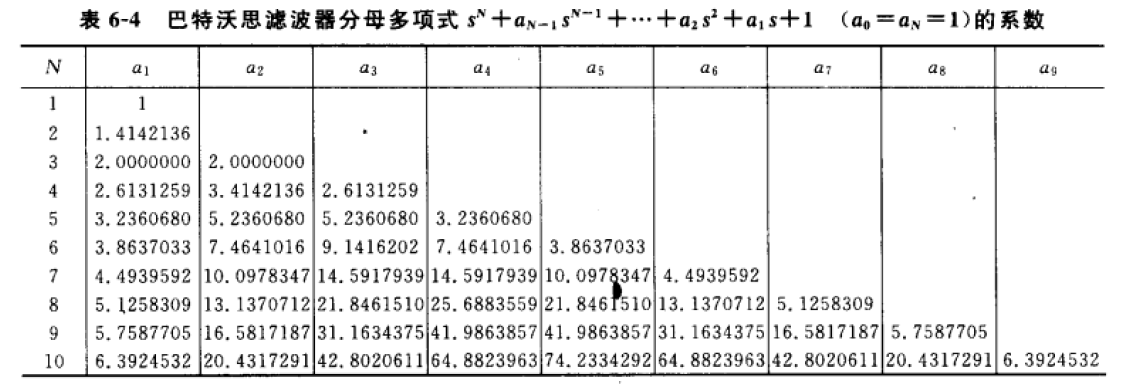意思是如果你按照表格里的参数，带入滤波器公式中，就能得到对于阶数的归一化滤波器，即截止频率为1弧度（$1/2\pi$hz）的滤波器。
例如我想得到一个截止频率为$1/2\pi$hz的二阶巴特沃斯滤波器，就把n=2的系数带入方程，可得
$H(s) = \frac{1}{s^2+1.414s+1}$
有了这个归一化滤波器，我们可以很容易的得到任意截止频率的滤波器，只需要另$s->\frac{s}{w_a}$就可以的带一个截止频率为wa弧度的滤波器。
$\frac{1}{\frac{s}{w_a}^2+1.414\frac{s}{w_a}+1}$
模拟与数字的关系
上面举例的模拟滤波器传递函数，目的是用来设计滤波电路，针对的是连续时间的模拟信号，组成元器件是电阻，电容，电感。
而数字滤波器实现方法是把滤波器所要完成的运算编成程序并让计算机执行,也就是采用在代码的形式。它面对的是离散时间的数字信号，是把输入序列通过一定的运算变换成输出序列。
有没有办法能把连续的模拟滤波器变成离散的数字滤波器？
显然是有的，而且有很多种，其中最常使用的一种叫做双线性变换
$s=\frac{2}{T_s}\frac{1-z^{-1}}{1+z^{-1}} =2f_s\frac{1-z^{-1}}{1+z^{-1}}$
把这个公式带入传递函数就可以得到一个z域的差分方程了。
但是如果我们直接使用双线性变换进行离散化之后，会发现转换前的模拟滤波器和转换后的数字滤波器的幅频响应曲线并不一样。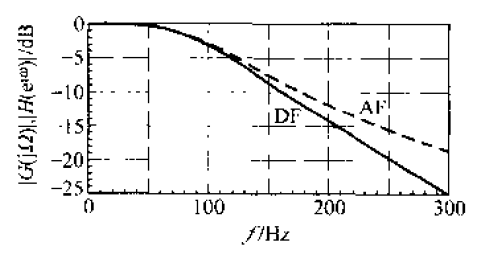可以看到数字滤波器曲线DF，远比模拟滤波器AF衰减的要快，也就是说如果模拟截止频率是10hz,那数字滤波器衰减更快，截止频率可以只9hz。
这是为什么呢？这是因为双线性变换中，数字截止角频率 $\omega_d$ 和模拟截止角频率 $\omega_a$ 的关系是非线性的。
所以在变换的时候我们需要找到s域与z域变换时频率变化的对应关系。
对于s域来说，在模拟截止频率为 $f_a$ 时有：
$s = jw_a = j*2\pi * f_a$
对于z域来说，在数字截止频率为fd的情况下，$z=e^{sT}$ 其中 $s=jw_d,w_d=2\pi f_d,T=1/f_s$，则有：
$z = e^{sT} = e^{j 2 \pi \frac{f_{d}}{f_{s}}}$
把这个截止频率为 $f_d$ 的带入双线性变换公式可以得到：
$s=2f_s\frac{1-z^{-1}}{1+z^{-1}}=2 f_{s} \frac{1-e^{-j 2 \pi \frac{f_{d}}{f_{s}}}}{1+e^{-j 2 \pi \frac{f_{d}}{f_{s}}}}=2 f_{s} \frac{e^{j 2 \pi \frac{f_{d}}{f_{s}}}-1}{e^{j 2 \pi \frac{f_{d}}{f_{s}}}+1}=j 2 f_{s} \tan \left(\frac{\pi f_{d}}{f_{s}}\right)$
所以
$j 2 \pi f_{a}=j 2 f_{s} \tan \left(\frac{\pi f_{d}}{f_{s}}\right)$
可以得到模拟截止频率与数字截止频率的关系
$f_a = \frac{f_s}{\pi}\tan(\frac{\pi f_d}{f_s})$
（补充证明过程根据欧拉公式）
$e^{i x}=\cos x+i \sin x$
$\frac{\mathrm{e}^{jA}-\mathrm{e}^{-jA}}{\mathrm{e}^{jA}+\mathrm{e}^{-jA}} = \frac{\cos(A)+j\sin(A)-(\cos(-A)+j\sin(-A))}{\cos(A)+j\sin(A)+(\cos(-A)+j\sin(-A))} = j\tan(A)$
$\frac{e^{jw}-1}{e^{jw}+1} = \frac{\mathrm{e}^{jw / 2}}{\mathrm{e}^{\mathrm{j} \omega / 2}} \frac{\left(\mathrm{e}^{\mathrm{j} \omega / 2}-\mathrm{e}^{-\mathrm{j} \omega / 2}\right)}{\left(\mathrm{e}^{\mathrm{j}\omega / 2}+\mathrm{e}^{-\mathrm{j} \omega / 2}\right)}=j\tan(\frac{\omega}{2})$
设计数字滤波器
有了离散方式和频率对应关系，就可以来设计我们需要的数字滤波器了。
设计数字滤波器分几步？

根据我们想要的数字滤波频率得到我们想要的模拟滤波器频率

根据期望的模拟截止频率，将滤波器去归一化。

进行双线性变换

写成代码

如果我期望的数字滤波器截止频率为fd,对应的模拟滤波器截止角频率为：
$w_a = 2\pi f_a = 2f_s\tan(\frac{\pi f_d}{f_s})$
去归一化只需要将模拟滤波器传递函数中的s进行如下替换即可
$s->\frac{s}{w_a}$
最后使用双线性变换离散化,把公式带入
$s=2f_s\frac{1-z^{-1}}{1+z^{-1}}$
所以最终的变换方式就是
$s->\frac{s}{w_a} = \frac{2f_s\frac{1-z^{-1}}{1+z^{-1}}}{2f_s\tan(\frac{\pi f_d}{f_s}) } = \frac{\frac{1-z^{-1}}{1+z^{-1}}}{\tan(\frac{\pi f_d}{f_s}) }$
ok,看起来有一点点复杂，没关系，举个例子
还是以我们的归一化二阶巴特沃斯低通滤波器公式
$H(s) = \frac{1}{s^2+1.414s+1}$
带入完整的变换可得
$H(z) = \frac{1}{{(\frac{ \frac{1-z^{-1}}{1+z^{-1}} } {\tan(\frac{\pi f_d}{f_s}) })}^2+1.414(\frac{ \frac{1-z^{-1}}{1+z^{-1}} } {\tan(\frac{\pi f_d}{f_s}) }))+1} = \frac{ (\tan(\frac{\pi f_d}{f_s}))^2 } {(\frac{1-z^{-1}}{1+z^{-1}})^2 + 1.414(\frac{1-z^{-1}}{1+z^{-1}})*(\tan(\frac{\pi f_d}{f_s})) + (\tan(\frac{\pi f_d}{f_s}))^2}$
为了避免过于复杂我们把常数$\tan(\frac{\pi f_d}{f_s})$重新命名为$\Omega_{new}$
$H(z)=\frac{Y(z)}{X(z)}=\frac{\left(1+z^{-1}\right)^{2} \Omega_{new}^{2}}{\left(1-z^{-1}\right)^{2}+1.414 \Omega_{new}\left(1-z^{-1}\right)\left(1+z^{-1}\right)+\Omega_{new}^{2}\left(1+z^{-1}\right)^{2}}$
$H(z)=\frac{Y(z)}{X(z)}=\frac{\Omega_{new}^{2}+2 \Omega_{new}^{2} z^{-1}+\Omega_{new}^{2} z^{-2}}{1-2 z^{-1}+z^{-2}+1.414 \Omega_{new}-1.414 \Omega_{new} z^{-2}+\Omega_{new}^{2}+2 \Omega_{new}^{2} z^{-1}+\Omega_{new}^{2} z^{-2}}$
$H(z)=\frac{Y(z)}{X(z)}=\frac{\Omega_{new}^{2}+2 \Omega_{new}^{2} z^{-1}+\Omega_{new}^{2} z^{-2}}{\left(1+1.414 \Omega_{new}+\Omega_{new}^{2}\right)+\left(2 \Omega_{new}^{2}-2\right) z^{-1}+\left(1-1.414 \Omega_{new}+\Omega_{new}^{2}\right)z^{-2}}$
到这里我们的化简就全部结束了，什么还是好复杂？那我再重新给他们起个名字吧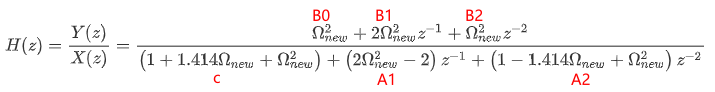令$B0=\Omega_{new}^{2}$,$B1=2\Omega_{new}^{2}$,$B2=\Omega_{new}^{2}$,
$c=1+1.414\Omega_{new}+\Omega_{new}^{2}$,$A1=2\Omega_{new}^{2}-2$,$A2=1-1.414\Omega_{new}+\Omega_{new}^{2}$
$H(z)=\frac{Y(z)}{X(z)}=\frac{B_0+B_1 z^{-1}+B_2 z^{-2}}{ c+A_1 z^{-1}+A_2z^{-2}}$
$Y(z)( c+A_1 z^{-1}+A_2z^{-2}) = X(z)(B_0+B_1 z^{-1}+B_2 z^{-2})$
$Y(z) = \frac{B_0}{c}X(z)+\frac{B_1}{c} X(z)z^{-1}+\frac{B_2}{c} X(z)z^{-2} - \frac{A_1}{c} Y(z)z^{-1} - \frac{A_2}{c}Y(z)z^{-2}$
$Y(z) = \frac{B_0}{c}X(z)+\frac{B_1}{c} X(z-1)+\frac{B_2}{c} X(z-2)- \frac{A_1}{c} Y(z-1) - \frac{A_2}{c}Y(z-2)$
再改写一下
$Y(z) = b0X(z)+b1 X(z-1)+b2 X(z-2)-a1Y(z-1)-a2Y(z-2)$
有没稍微熟悉一点？这不就是书上的公式吗？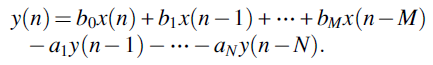根据我们之前的推导，这个差分方程的系数只跟数字截止频率 $f_d$，和采样频率 $f_s$ 有关。
$\Omega_{new}=\tan(\frac{\pi f_d}{f_s})$
$c=1+1.414\Omega_{new}+\Omega_{new}^{2}$
$b0=B0/c=\Omega_{new}^{2}/c$,$b1=B1/c=2\Omega_{new}^{2}/c$,$b2=B2/c=\Omega_{new}^{2}/c$,
$a1=A1=(2\Omega_{new}^{2}-2)/c$,$A2=(1-1.414\Omega_{new}+\Omega_{new}^{2})/c$
看看APM和PX4怎么用程序怎么计算这些些系数呢？（跟我们的推导一模一样）系数有了那这样的差分方程怎么写成代码呢？转换成差分方程后，下标n就代表当前值，下标n-1代表这个数是上个周期的值，n-2代表这个数延时了两周期。
所以方程写在代码里就是：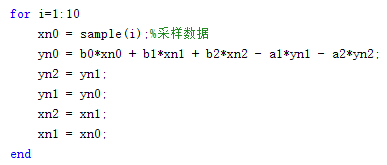这种形式有个名称叫做直接I型，我们可以将它化成框图形式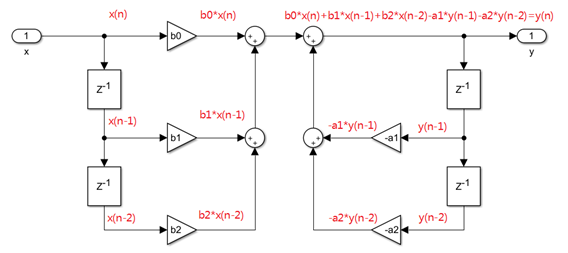从框图中可以直观的看见使用了4个z^-1（延时模块）,从代码里也可以看见，我们需要保存4个过去的值（x(n-2),x(n-1),y(n-2),y(n-1)）,
一个二阶滤波器需要4个延时模块我们能不能化简一下呢？
我们观察上图的直接I型滤波器，可以看成是两个较小系统串联而成的系统，那么，我们将其调整一下位置，得到就像下图一样的一个新的系统。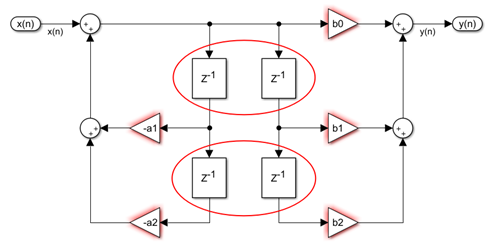仔细观察其实我们可以共用延时模块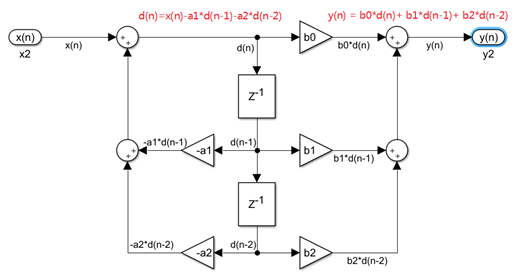这样我们只需要使用两个延时模块就行，写成代码如下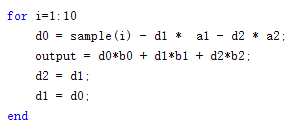这样我们在代码里只需要保存两个数据就行了，在看看源码，是不是一模一样？到这里我们才算完成了一个滤波器设计，完整的称呼应该叫做 二阶巴特沃斯低通滤波器使用双线性变换法的IIR数字滤波器实现。
什么是IIR？什么是巴特沃斯？
IIR 是一种实现滤波器的方法，对数字滤波器，从实现方法上有IIR和FIR滤波器之分，他们分别对应的是不同的滤波器结构
IIR实现的最终效果就是我们刚才设计的过程对应的通用转移函数结构如下
$H(z)=\frac{\sum_{r=0}^{M} b_{r} z^{r}}{1+\sum_{k=1}^{N} a_{k} z^{-k}}$
如果使用FIR的方式实现，对应的转移函数形式如下
$H(z)=\sum_{n=0}^{N-1} h(n) z^{-n}$
FIR滤波器可以对给定的率特性直接进行设计，而IIR滤波器目前最通用的方法是利用已经很成熟的模拟滤波器的设计方法来进行设计。
所以IIR可以利用不同的模拟滤波器来设计，
而模拟滤波器又有Butterworth滤波器、Chebyshev(I型、Il型)滤波器、椭圆滤波器等不同的设计方法，
对应不同的幅度平方函数，以巴特沃斯滤波器为例：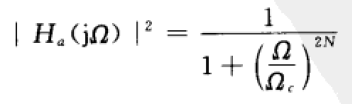使用这种函数需要进行一些零极点配置，才能得到我们想要的传递函数，好在模拟滤波器设计非常成熟，有各种表格，我们查表就能直接得到对应的滤波器传递函数。
而双线性变换是离散化的一种方法，通过这种方式离散可以直接得到IIR的结构。
谁能想到一个二阶滤波器而已，不过十几行代码，里面有这么多数字信号处理的知识呢？OK，今天就讲这么多，我是zing，我们下期再见。


展开全文iir滤波器
• 标准形式一边是关于u关于x的二阶导数，一边是关于x的函数。 求解思路 我们现在用有限差分法解下面一个简单的一维弦震动问题 −u′′(x)=16π2sin(4πx) 其中边值条件为u(0)=0,u(1)=0. 根据我们之前的内容，可以将解...
• 为了更适合于数字图像处理，将该方程表示为离散形式.另外，拉普拉斯算子还可以表示成模板的形式，以便更好编程需要。这种简单的锐化方法既可以产生拉普拉斯锐化处理的效果，同时又能保留背景信息，将原始图像叠加到...计算机视觉 图像分析 Laplace算子
• 马尔萨斯人口模型：差分方程—>微分方程 但应用比较少。 改进：考虑剩余环境容纳量，logistic模型 再改进… 负密度依赖：密度越大，增长越慢 弦振动、热传导方程 微分方程的解法 1.可分离变量的 2.一阶线性常微分...数学建模 数学
• 以具有倾斜对称轴的横向各向同性(Titled Transverse Isotropic,TTI)介质中纯准P波二阶方程为基础,通过引入辅助波场实现了方程的降阶,推导出了TTI介质纯准P波一阶压力-速度方程,并利用旋转交错网格高阶有限差分方法...
• 上一篇文章写了正方形网格的情形，实际计算中常常用到非等距矩形网格...方程左边是f，右边是原函数二阶导数的差分形式。将u[i,j]移到左边，除掉系数就得到u的方程组。 代码如下 import numpy as np import matplo...
• 问题描述  在用差分法求解二阶常微分方程的边值问题、热传导问题及三次样条插值函数的求解问题中，都会遇到下面形式的阶数较高的三对角方程组AX=fAX=f，即 ⎡⎣⎢⎢⎢⎢⎢⎢⎢b1a2⋱c1b2an−1⋱c2bn−1⋱ancn−1...
• 二阶差分（也叫递推）式a*f(n+2)+b*f(n+1)+c*f(n)=0中，为了求出一阶差分式，我们总希望将原式子变形成 f(n+2)-x1*f(n+1)=x2*( f(n+1)-x1*f(n) )的形式，因为如果有这样的常数x1，x2使式子成立，那么，数列{f(n+1...c
• 二阶常系数线性差分方程齐次差分方程二阶常系数线性差分方程的一般形式为： yn+ayn+1+byn=f(n)，n=0，1，2，....(1−1)y_n+ay_{n+1}+by_n=f(n)， n=0， 1， 2，....(1-1) 其中a，b为已知常数，且b≠0，f(n)为已知...斐波那契数列 时间复杂度
• 18.1具有常系数和常数项的二阶线性差分方程 18.2萨缪尔森乘数一加速数相互作用模型 18.3离散时间条件下的通货膨胀与失业 18.4推广到可变项和高阶方程 第19章　联立微分方程与差分方程 19.1动态方程组的起源 19.2解...
• 非线性耦合系统的特征差分方法，袁益让，，对多层非线性渗流方程的耦合系统提出适合并行计算的二阶和一阶特征分数步差分格式.利用变分形式,能量方法,二维和三维网格配套,双�
• 时域中常用的数学模型有微分方程、差分方程和状态方程；复数域中有传递函数、结构图；频域中有频率特性等。 本次课程设计研究的是I型二阶系统的分析和设计，主要是培养学生统筹运用自动控制原理课程中所学的理论知识...
• 使用有限差分法 得出下一个时间n+1在方格i上的数值 泊松方程 p是一个标量，比如说压力。b是一个源，或者说是b的初始分布函数 然后记住二阶导长这样 转换方程，写成机器能看懂的形式 最终得到 The...
• <br />一种特殊情况 特别地，对于二阶常系数线性奇次微分方程（方程的右边的值为零），解的情况与差分方程基本一样，而对于用微分算子解答的情况，方程的右边的值不为零。 同样地，这里来证明下有重...
• peEllip5m 用工字型差分格式解拉普拉斯方程 peHypbYF 用迎风格式解对流方程 peHypbLax 用拉克斯-弗里德里希斯格式解对流方程 peHypbLaxW 用拉克斯-温德洛夫格式解对流方程 peHypbBW 用比姆-沃明格式解对流方程 ...
• ## MATLAB常用算法

热门讨论 2010-04-05 10:34:28
peEllip5m 用工字型差分格式解拉普拉斯方程 peHypbYF 用迎风格式解对流方程 peHypbLax 用拉克斯-弗里德里希斯格式解对流方程 peHypbLaxW 用拉克斯-温德洛夫格式解对流方程 peHypbBW 用比姆-沃明格式解对流方程 ...MATLAB 算法
• 按照传递函数的微分方程的阶数：一阶、二阶、……高阶。 几种滤波器的幅频特性曲线 上图中（a)低通滤波器、（b）高通滤波器、（c)带通滤波器、（d）带阻滤波器 理想滤波器的幅频特性： 几种按照“最
• 9·12 偏微分方程差分解法 第十章 微分几何学 10·1 平面曲线 10·2 空间曲线 10·3 曲面的参数表示 10·4 曲面的第一、第二基本形式 10·5 曲面上的曲率 10·6 曲面的球面表示.第三基本形式 10·7 直纹曲面.可展...
• 4·3 二阶微分方程的解法 第十三章 概率·统计 1.概率 1·1 概率的定义 1·2 概率计算的基本定理 2.统计 2·1 频数分布及频数分布图 2·2 相关分析 2·3 总体与样本 2·4 期望值 2·5 统计的假设检验 第十四章 初等...
• 4·3 二阶微分方程的解法 第十三章 概率·统计 1.概率 1·1 概率的定义 1·2 概率计算的基本定理 2.统计 2·1 频数分布及频数分布图 2·2 相关分析 2·3 总体与样本 2·4 期望值 2·5 统计的假设检验 第十四章 初等...
• 其他功能包括：RC时间常数计算，电阻串、并联计算，电阻反向串、并联计算，变压器计算器，LM317三端稳压器件计算，TL431精密基准电压计算，RC无源滤波电路计算，二阶有源滤波电路计算 ，差分低通滤波电路计算，多层...
• 9.差分二阶LPF 这个电路常用于差分输出的DAC的LPF电路(默认使用最佳Q值计算).使用方法请参考第6点. 10.多层电感 此功能用于空心多层电感的估算(如音箱分频器的空心电感).只需输入所要的电感量和直流电阻(直流电阻...
• 11.差分二阶LPF 这个电路常用于差分输出的DAC的LPF电路(默认使用最佳Q值计算).使用方法请参考第6点. 12.多层电感 此功能用于空心多层电感的估算(如音箱分频器的空心电感).只需输入所要的电感量和直流电阻(直流...
• 11.差分二阶LPF 这个电路常用于差分输出的DAC的LPF电路(默认使用最佳Q值计算).使用方法请参考第6点. 12.多层电感 此功能用于空心多层电感的估算(如音箱分频器的空心电感).只需输入所要的电感量和直流电阻(直流...